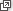## Content

### Linear equations and inequalities

Many problems that arise in the applications of mathematics lead naturally to equations. Equations in which the unknown only occurs to the first power are called linear equations. Thus

$2x-4=6 \qquad \text{and} \qquad \frac{5x}{2}-7= 4x+\frac{1}{2}$ are examples of linear equations, whereas $x^2-5x+ 6=0 \qquad \text{and} \qquad \frac{2x}{x-1}=\frac{x-3}{2x+5}$ are not linear, but are examples of quadratic equations which will be dealt with later. Equations such as $x^3 -3x^2 +6x -4 = 0$ are called polynomial equations, and equations such as $e^x=x-3$

are often referred to as transcendental equations. With some exceptions, such transcendental equations are generally not dealt with in secondary school mathematics.

It is important to distinguish clearly between identities and equations. An identity is a statement that is true for (almost) all values of the pronumeral. For example,

$(x+1)^2=x^2+2x+1$

is an identity, since when any real number is substituted for $$x$$ a true statement results. An equation is generally only true for certain values of the pronumeral. Thus, $$3x-2=10$$ only yields a true statement when $$x$$ takes the value 4. This is called the solution of the equation.

Linear equations only have (at most) one solution. Can you write down a linear equation with no solution?

#### Linear equations

The various types of linear equations and the various strategies to solve them are dealt with at length in the module Linear equations (Years 7–8), and so we will only quickly revise some of these ideas here via two examples. The basic rule throughout is that whatever you do to one side of the equation you must also do to the other.

#### Example

Solve

$\frac{5x-3}{2}=6.$

#### Solution

\begin{align*} \frac{5x-3}{2}&=6\\ 5x-3&=12\\ 5x&=15\\ x&=3. \end{align*}

#### Example

Solve

$\frac{2x+1}{4}=\frac{3x-1}{7}.$

#### Solution

\begin{align*} \frac{2x+1}{4}&=\frac{3x-1}{7}\\ 7(2x+1)&=4(3x-1)\\ 14x+7&=12x-4\\ 2x&=-11\\ x&=-\frac{11}{2}. \end{align*}

#### Linear inequalities

A linear inequality resembles in form an equation, but with the equal sign replaced by an inequality symbol. The solution of a linear inequality is generally a range of values, rather than one specific value. Such inequalities arise naturally in problems involving words such as 'at least' or 'at most'.

To solve an inequality we use the same procedures as we used when solving linear equations, with the modification that when an inequality is multiplied or divided by a negative number, the inequality is reversed.

#### Example

Solve

$\frac{1-2x}{3}\leq 9.$

#### Solution

\begin{align*} \frac{1-2x}{3} &\leq 9\\ 1-2x &\leq 27\\ -2x &\leq 26\\ x &\geq -13. \end{align*}

Inequalities also arise when we examine the domain of certain functions. Hence it is important that students are familiar with these before studying functions.

Next page - Content - Quadratic equations